# Simple Urban Energy Balance Model for Mesoscale Simulation (SUMM)

<- Back to homepage ( English / Japanese )

## Introduction

Urban surface geometry has a large, but complex, influence on urban meteorology. Unfortunately, explicit consideration of buildings into weather simulations using computational fluid dynamic methods is quite time-consuming and thus is still unrealistic for practical applications. An alternative approach has been to develop simple urban energy balance models for use in mesoscale simulations (Arnfield, 1982; Masson, 2000; Kusaka et al., 2001; Martilli et al., 2002; Sailor and Fan, 2002). These models generally assume two-dimensional (2-D) infinite street canyons mainly because it allows one to treat radiation with analytic theory. To overcome the restriction of a 2-D radiation scheme, we have recently developed a simple theoretical radiation scheme applicable for three-dimensional (3-D) rectangular obstacles arrays (Kanda et al., 2005). We propose a new simple urban energy balance model for mesoscale simulations (SUMM). The SUMM consists of a 3-D theoretical radiation scheme (Kanda et al., 2005) and the conventional heat transfer expression that uses a network of resistances (Masson, 2000; Kusaka et al., 2001). The present model allows one to readily calculate the energy balance and surface temperature at each face of the urban canopy (i.e., roof, floor, and four vertical walls) without time-consuming iterations.

## Technology

### 1. Geometry

The urban surface layer is simply divided into vegetated (Aveg) and non-vegetated (urban; 1-Aveg) fractions.  Each energy flux is calculated by the simple areal weighting of fluxes from vegetated and urban fractions.  In the urban fraction, SUMM explicitly resolves the three-dimensional surface geometry of the urban fraction.  Each building is regularly arranged in square horizontal cross-sections.  Such three-dimensional surface geometry is uniquely identified by two non-dimensional geometric parameters, i.e., the plane area index and the frontal area index, and the average building height.### 2. 3-D theoretical ratiation scheme

Without using time-consuming iterations or statistical approaches, we calculated the view factors of the faces, the complicated sunlit-shadow distributions, and the resulting canopy albedo for any time and location. The simple theoretical radiation scheme developed here can be applied to regular building arrays for any location and time. The outstanding point of this scheme is the high accuracy without any time-consuming iteration. Previous 3-D radiation models, such as Monte-Carlo simulation (Kondo et al., 2001) and finite difference (Kawai and Kanda, 2003), require significant computational-time even for the simple buildings arrays treated here.

 Direct beam distribution Single building case Building array case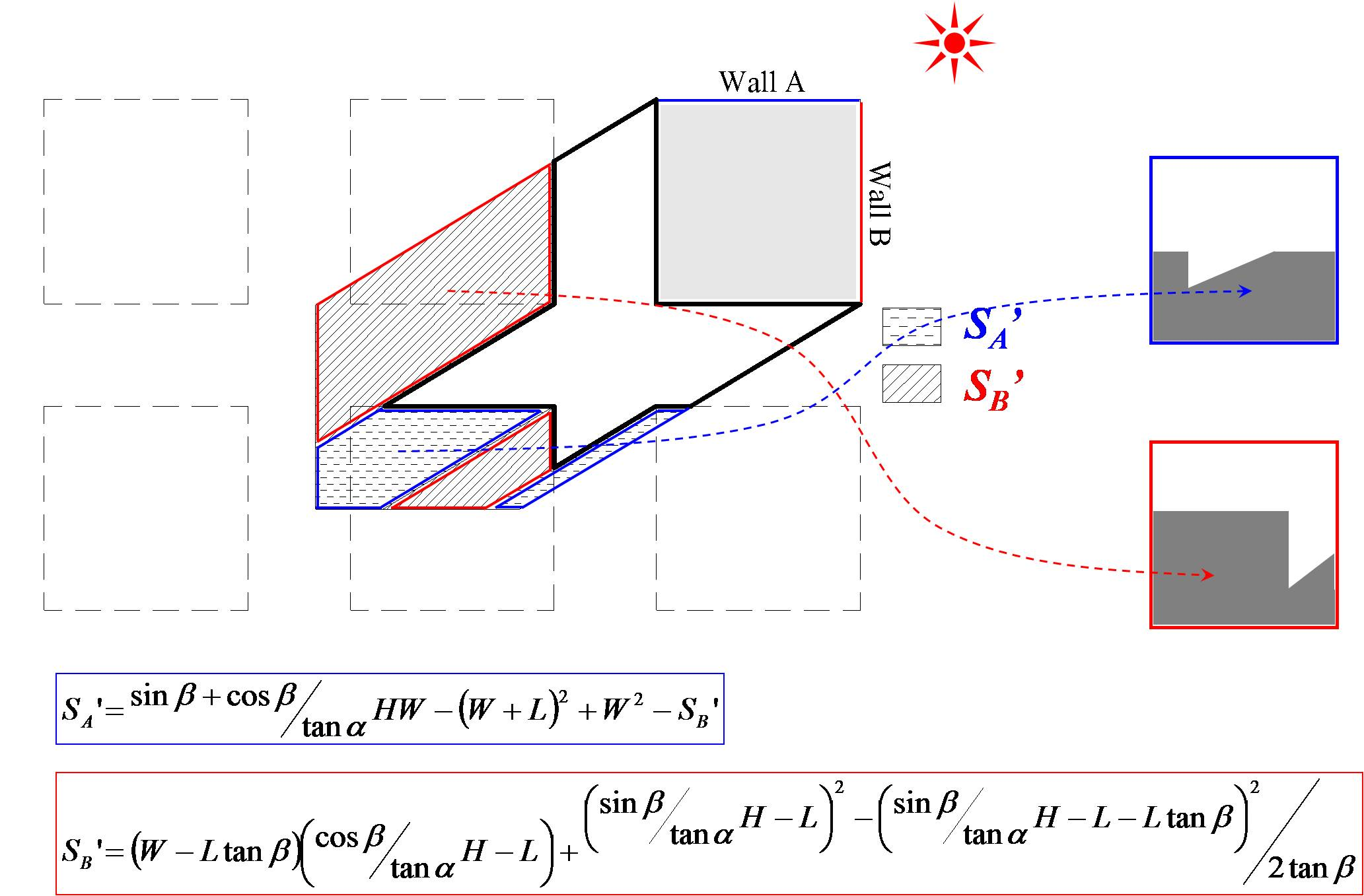Total 9 cases (plane aspect ratio > 0.25)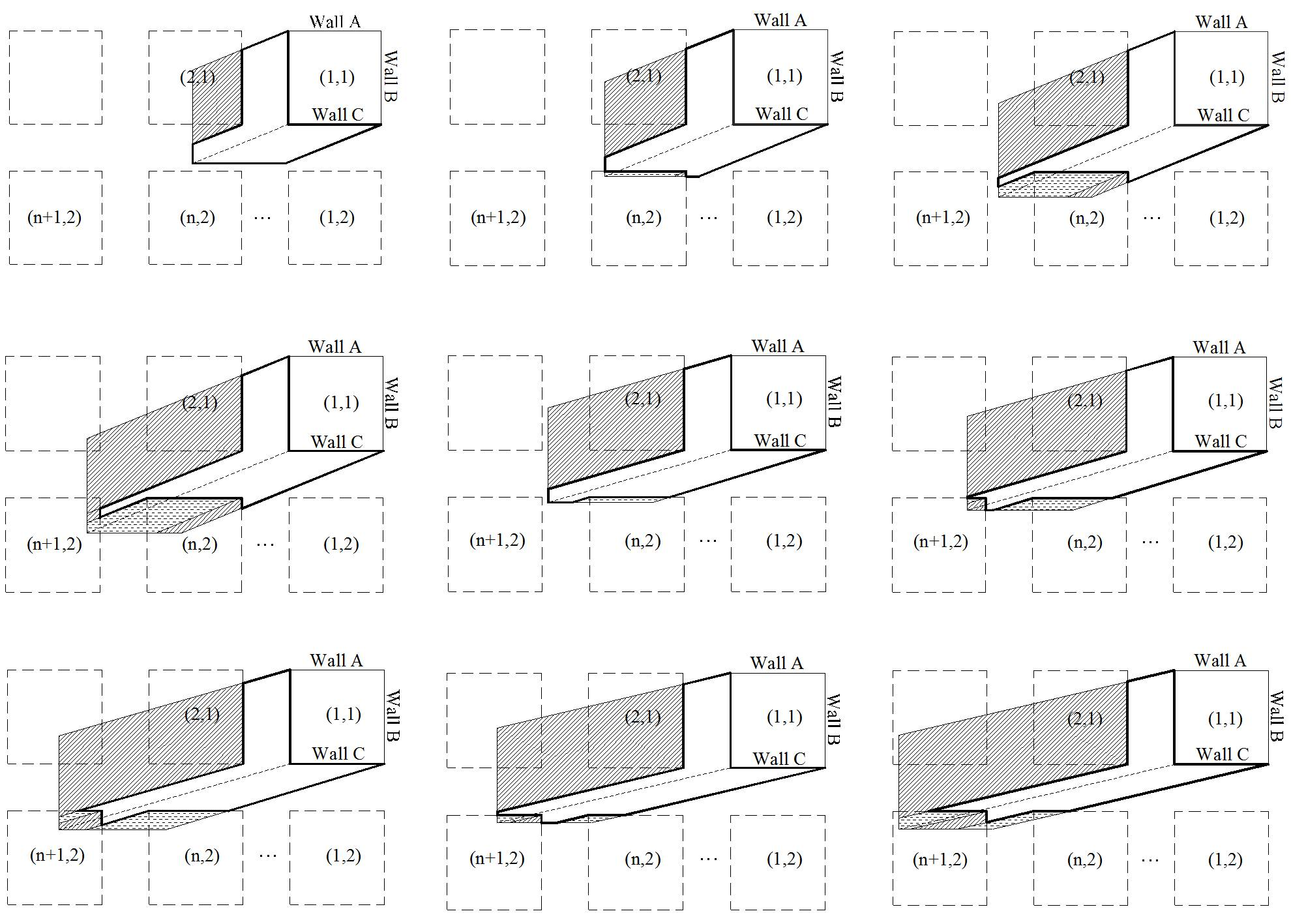### 3. Sensible and latent heat fluxes

The sensible and latent heat fluxes of the six faces are calculated using a network of resistance formulation similar to other simple urban canopy models.  As is well known, the parameterization of bulk transfer coefficient of local faces (CH(i)) is a key to the formulation.  However, CH(i) is currently difficult to arrange in a simple formulation.  Monin-Obukhov similarity theory (MOST) is valid only for the whole surface layer and the application of MOST to a local surface is physically incorrect.  SUMM uses a ‘top-down’ approach to determine CH(i).  First, the surface layer bulk transfer coefficient (CH) is determined by the MOST framework.  Second, CH is distributed to lacal values of CH(i) using relative values of CH(i).

 Top-down parameterization for CH(i)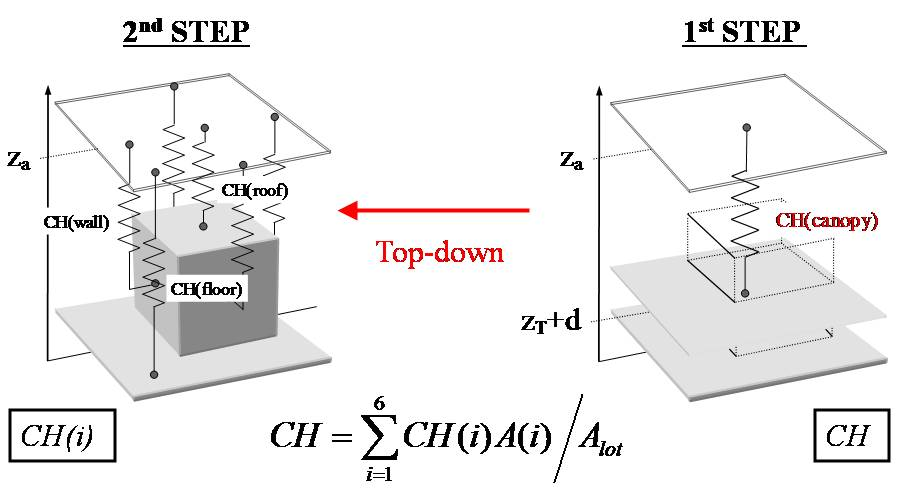Parameterization of kB-1 with vegetation effect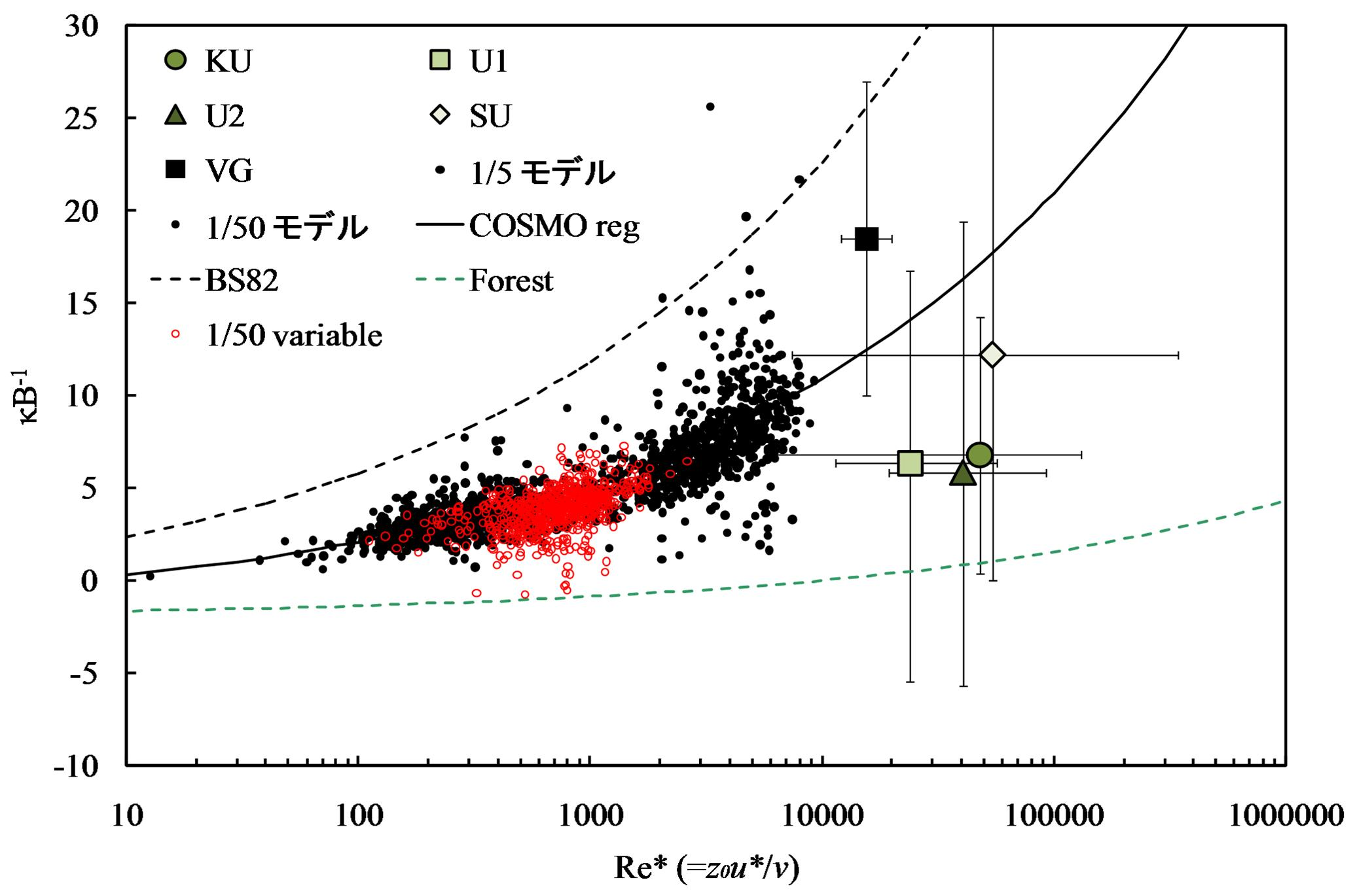### 4. Interior temperature prediction

The latest version of SUMM have the option of indoor temperature prediction including architectural factors such as air-conditioner scenario, glazing, and ventilation.

## Input/Ooutput data

### 1. Meteorological Forcing

 Unit 1 Downward shortwave radiation (direct and diffuse components) W m-2 2 Downward longwave radiatn W m-2 3 Wind velocity at reference height m s-1 4 Temperature at reference height K 5 Specific humidity at reference height kg/kg

### 2. Input parameters

 Unit Default value Location 1 Longitude - - 2 Latitude - - Geometrical parameters 1 Plane aspect ratio - - 2 Frontal aspect ratio - - 3 Plane area vegetation ratio - - 4 Street direction deg 0 5 Building height m - 6 Vegetation height m 8 7 Wall thichness m 0.25 8 Window fraction on a vertical wall - 0.3 Radiative parameters 1 Facet albedo of direct shortwave radiation (Urban fraction) - 0.15 2 Albedo of vegetation fraction - 0.05 3 Facet emissivity (Urban fraction) - 0.9 Thermal parameters 1 Volumetric heat capacity (Urban fraction) MJ m-3 K-1 2.5 2 Thermal conductivity (Urban fraction) W-1 m-1 K-1 1.01 Aerodynamic parameters 1 Natural logarizm of the ratio of roughness length for momentum to heat (kB-1=z0/zT) - Equation (*) 2 Roughness length for momentum (Vegetation fraction) (z0/H) - 0.3 3 CH(i)/CH(roof) - 1

## Results

Basic performance of SUMM (energy balance, surface temperature, and interior temperature) was evaluated using COSMO data, and then applied to the full-scale fields (three urban sites in two cities) with different surface geometry, location, and plane area vegetation ratio.  Examples of the results are shown below. For all of the four study sites, SUMM successfully simulated the surface layer energy fluxes, surface temperature, and interior temperature.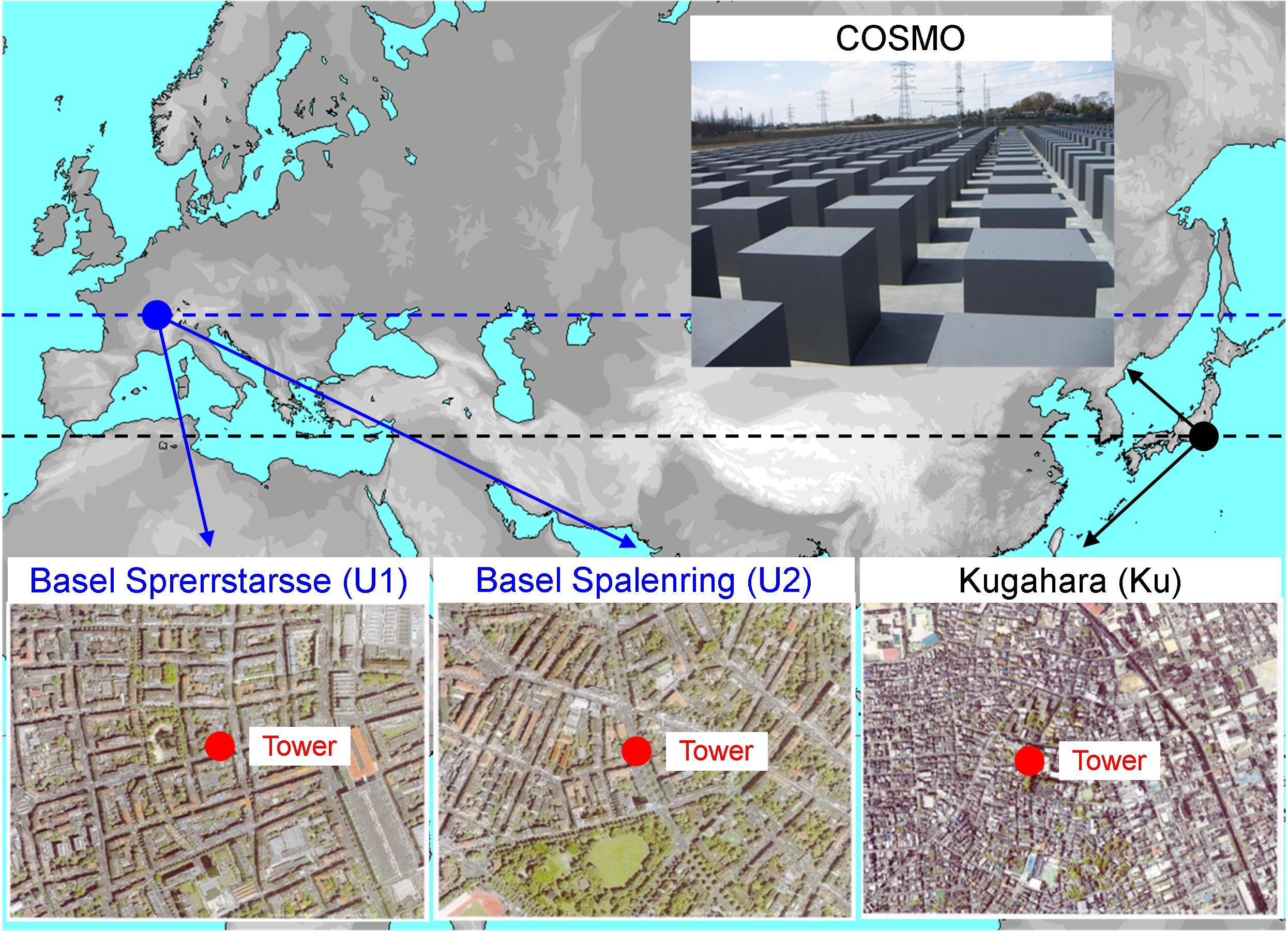Site Location Plane area vegetation ratio Plane aspect ratio Complete aspect ratio COSMO 36ｰ01'N, 139ｰ42'E 0.00 0.25 2.00 Kugahara 35ｰ34'N, 139ｰ41'E 0.21 0.33 2.80 Basel Sprerrstarsse (U1) 47ｰ34'N, 7ｰ35'E 0.16 0.54 1.92 Basel Spalenring (U2) 47ｰ33'N, 7ｰ34'E 0.31 0.37 1.75

[References]

1.  Moriwaki, R., and M. Kanda, 2004: Seasonal and diurnal fluxes of radiation, heat, water vapor and CO2 over a suburban area. J. Appl. Meteor., 43, 1700-1710.
2.  Christen, A., and R. Vogt, 2004: Energy and radiation balance of a central European city. Int. J. Climatol. 24: 1395-1421.

### 1. Results - COSMO

 Energy balance (winter) Energy balance (summer)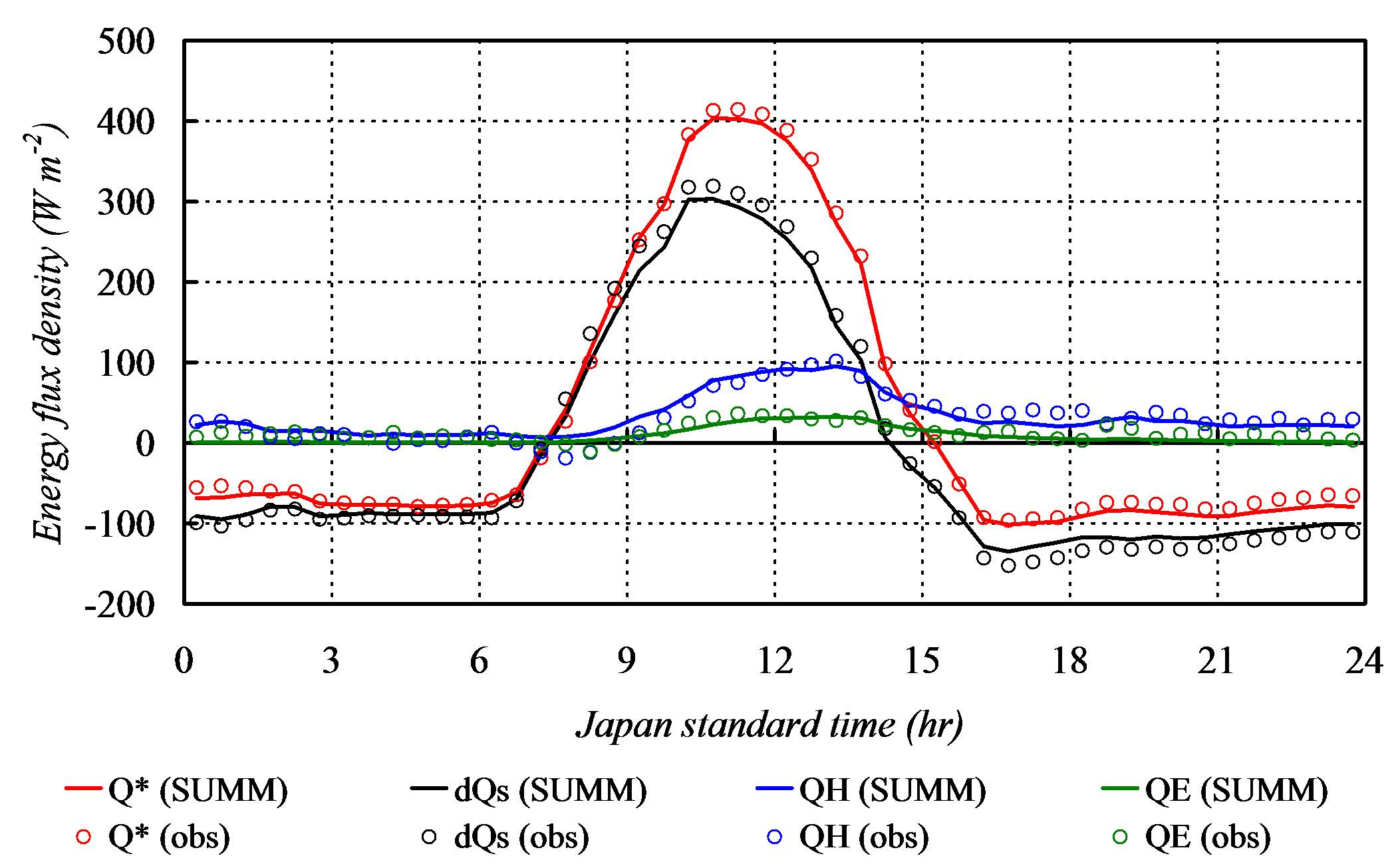Surface and interior temperature (winter) Surface and interior temperature (summer)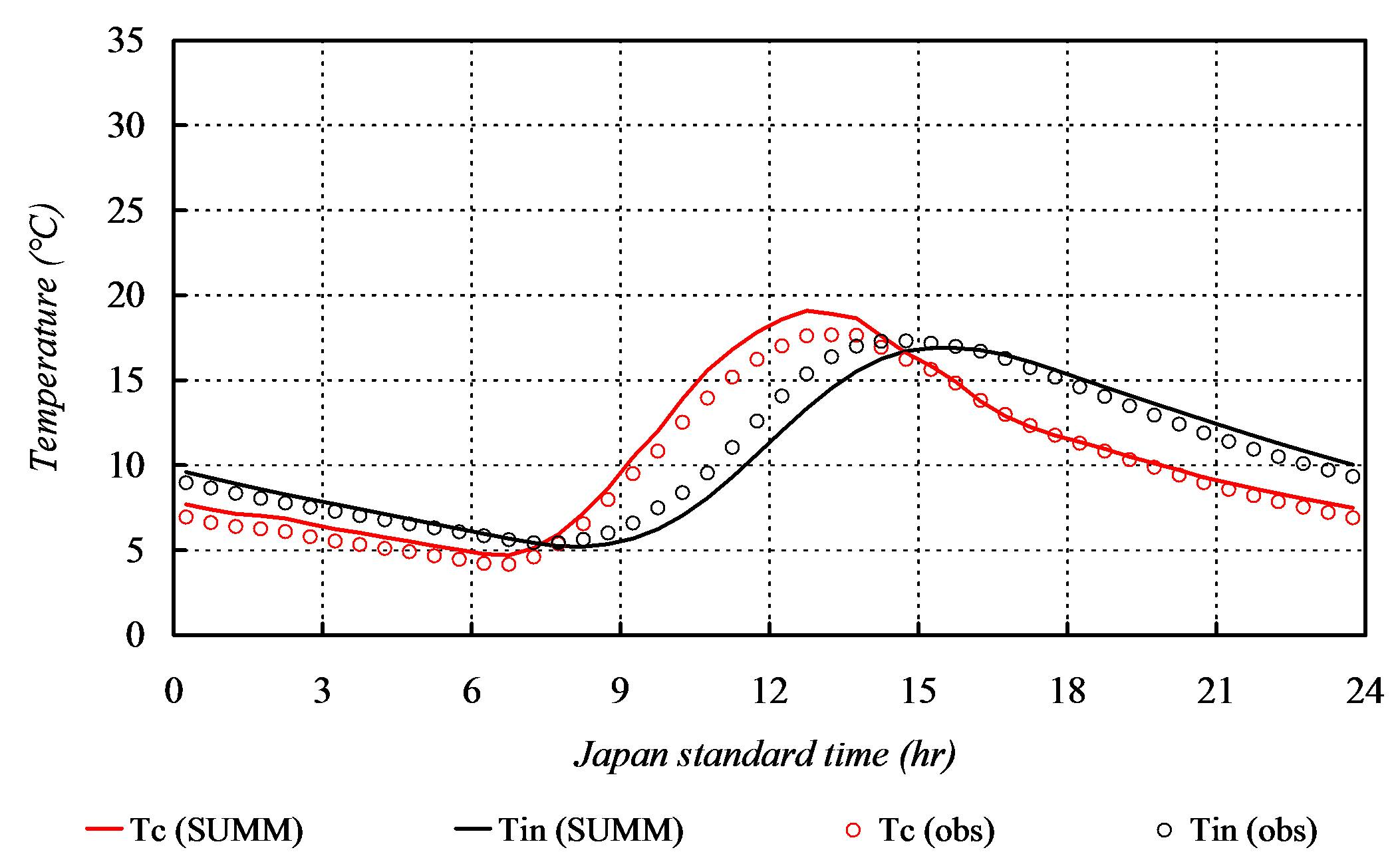### 2. Results (summer) - Full-scale fields

 Energy balance (Basel U1) Energy balance (Basel U2) Energy balance (Kugahara)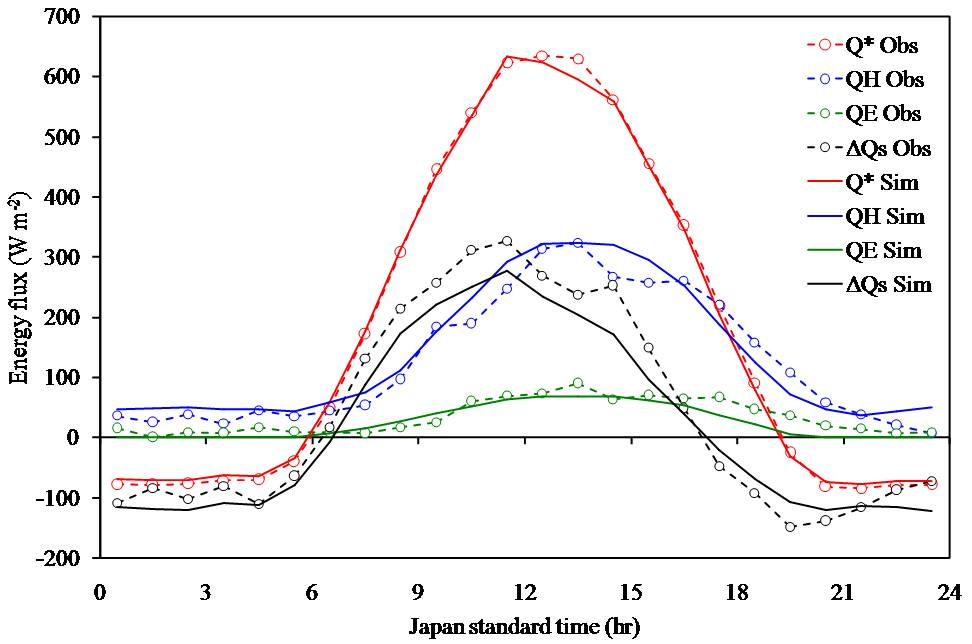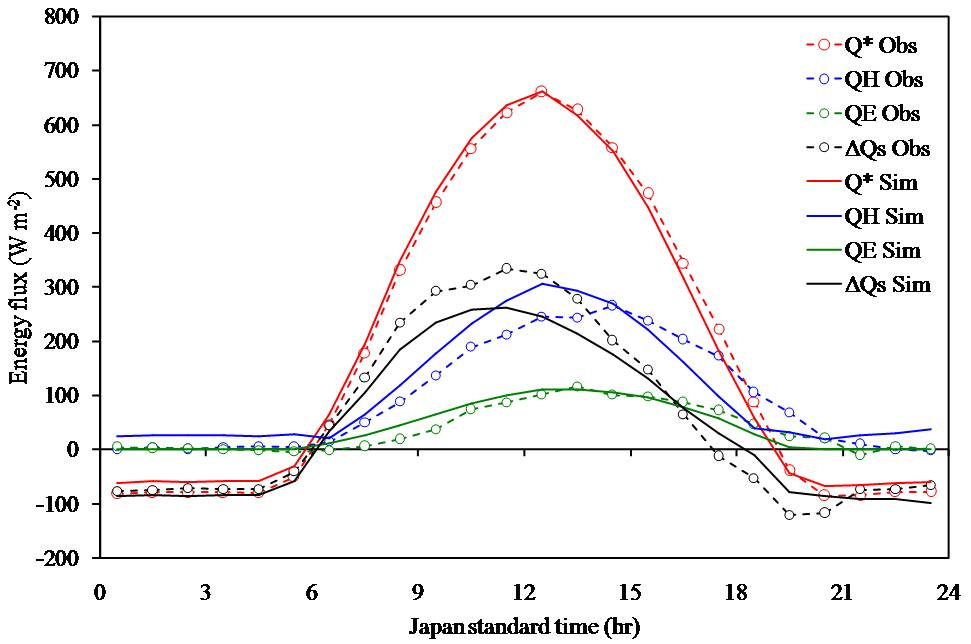Radiative temperature (Basel U1) Radiative temperature ( Basel U2) Radiative temperature (Kugahara)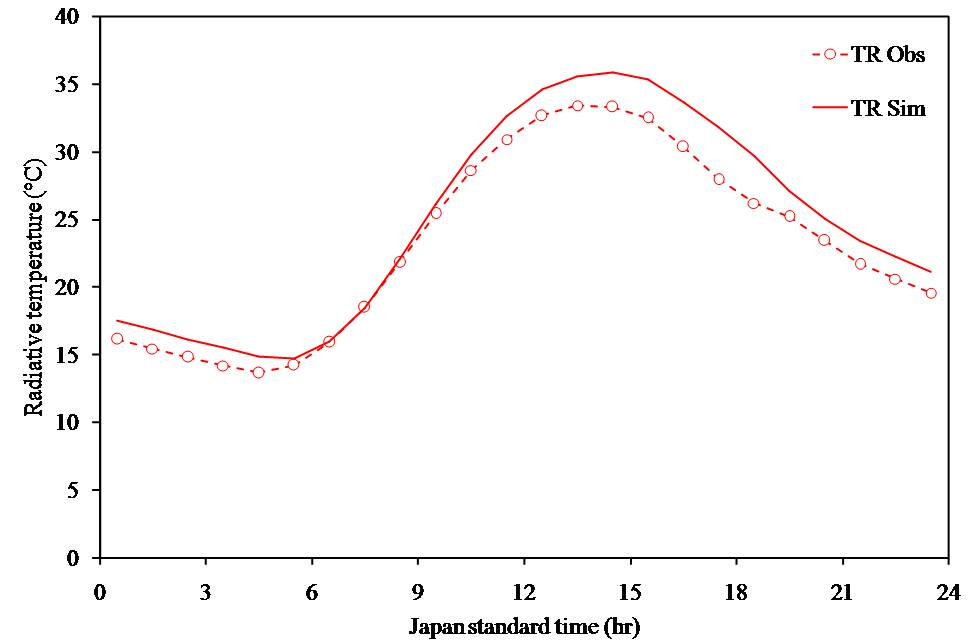## Publications

 Title Author(s) Journal Year Vol, pp 1 A Simple Theoretical Radiation Scheme for Regular Building Arrays Kanda M., Kawai T., Nakagawa K. Boundary-Layer Meteology 2005 114: 71-90 2 Simple Energy Balance Model for Regular Building Arrays Kanda M., Kawai T., Kanega M., Moriwaki R., Narita K., Hagishima A. Boundary-Layer Meteology 2005 116: 423-443 3 Roughness Rength for Momentum and Heat Derived from Outdoor Urban Scale Models Kanda M., Kanega M., Kawai T., Sugawara H., Moriwaki R. Journal of Applied Meteology and Climatology 2007 46: 1067-1079 4 Validation of a Numerical Model for Urban Energy-Exchange Using Outdoor Scale Model Measurements Kawai T., Kanda M., Narita K., Hagishima A. Interntional Journal of Climatology 2007 27: 1931-1942 5 Evaluation of a Simple Urban Energy Balance Model Using One-Year Observed Fluxes from Two Different Cities Kawai T., Mohammad K.R., Kanda M. Journal of Applied Meteology and Climatology 2007 Submitted